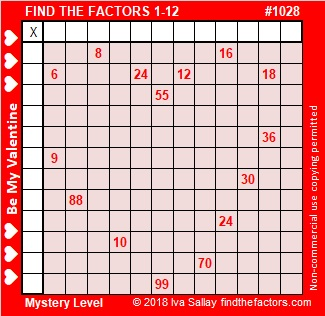# 1028 A Valentine Mystery

Valentine’s Day is almost here so I’ve made three Valentine related puzzles this week. I’ve labeled all of three of them Mystery Level because you might find some of them to be difficult. Use logic and an ordinary 12 × 12 multiplication table. I promise that each one of them can be solved, and I hope that you LOVE working on them! There will be some easier puzzles later on in the week.Print the puzzles or type the solution in this excel file: 12 factors 1028-1034

Let me tell you a little about the number 1028:

32² + 2² = 1028 so 1028 is the hypotenuse of a Pythagorean triple:
128-1020-1028 which is 4 times (32-255-257) and can be calculated from 2(32)(2), 32² – 2², 32² + 2²

1028 is a palindrome when it is written in a couple of different bases:
404 in BASE 16 because 4(16²) + 4(1) = 4(257) = 1028
2G2 in BASE 19 (G is 16 base 10) because 2(19²) + 16(19) + 2(1) = 1028

• 1028 is a composite number.
• Prime factorization: 1028 = 2 × 2 × 257, which can be written 1028 = 2² × 257
• The exponents in the prime factorization are 2 and 1. Adding one to each and multiplying we get (2 + 1)(1 + 1) = 3 × 2  = 6. Therefore 1028 has exactly 6 factors.
• Factors of 1028: 1, 2, 4, 257, 514, 1028
• Factor pairs: 1028 = 1 × 1028, 2 × 514, or 4 × 257
• Taking the factor pair with the largest square number factor, we get √1028 = (√4)(√257) = 2√257 ≈ 32.062439